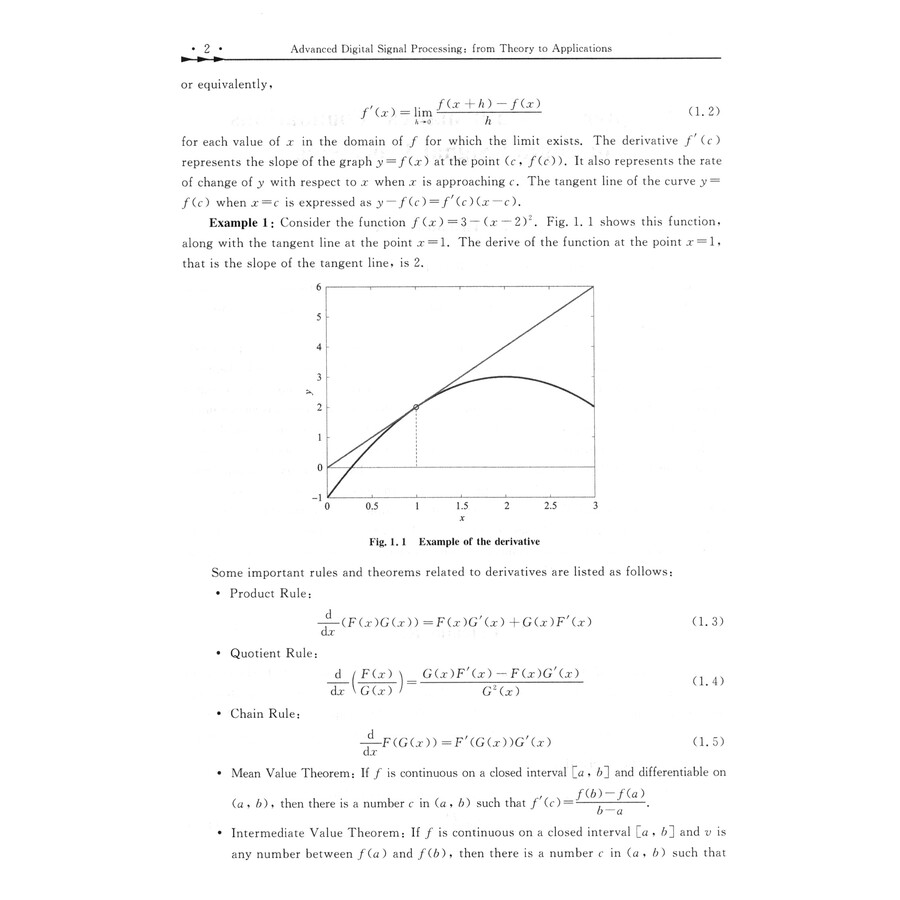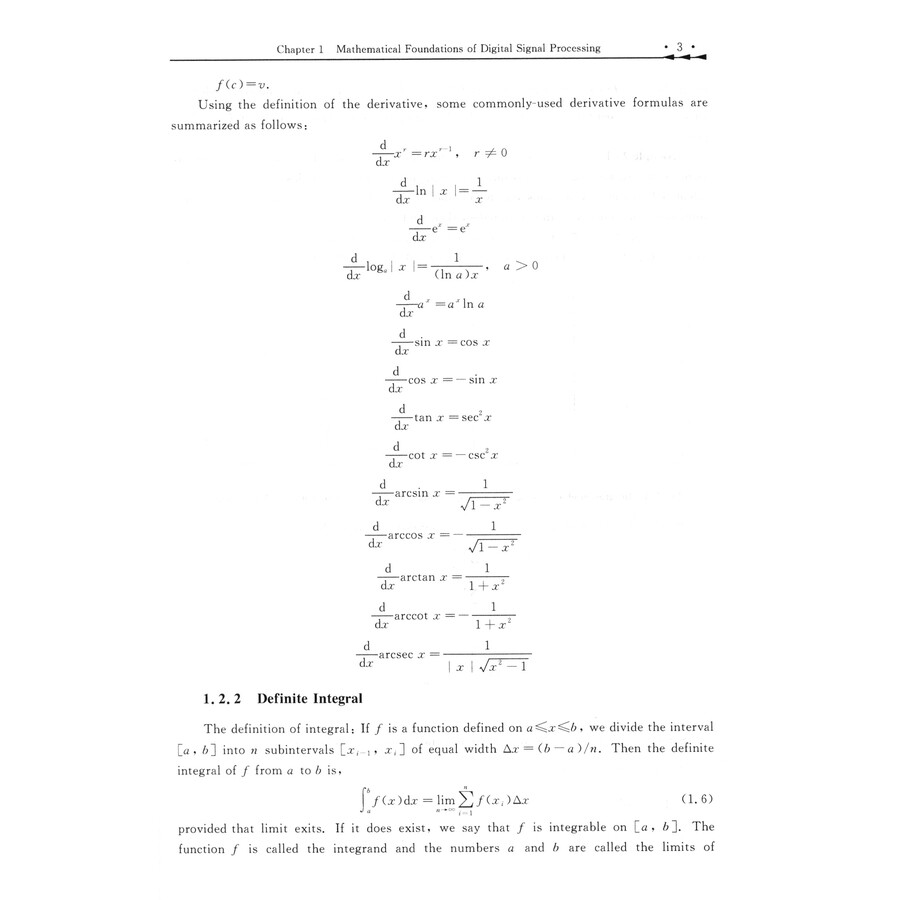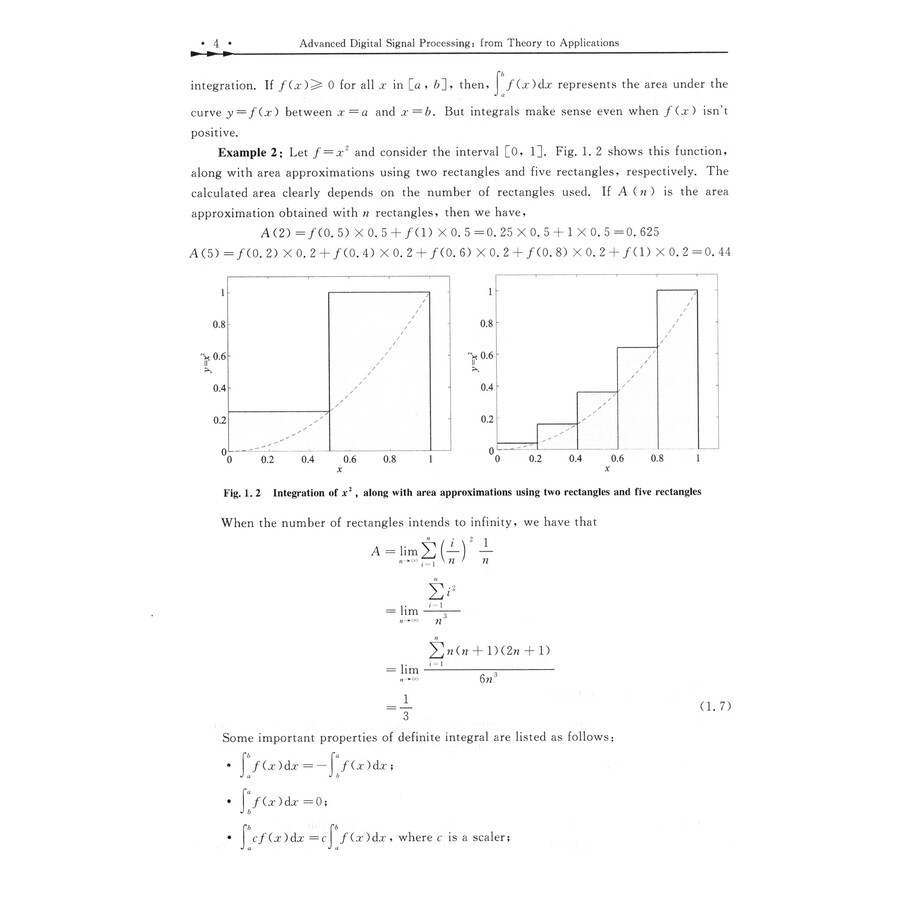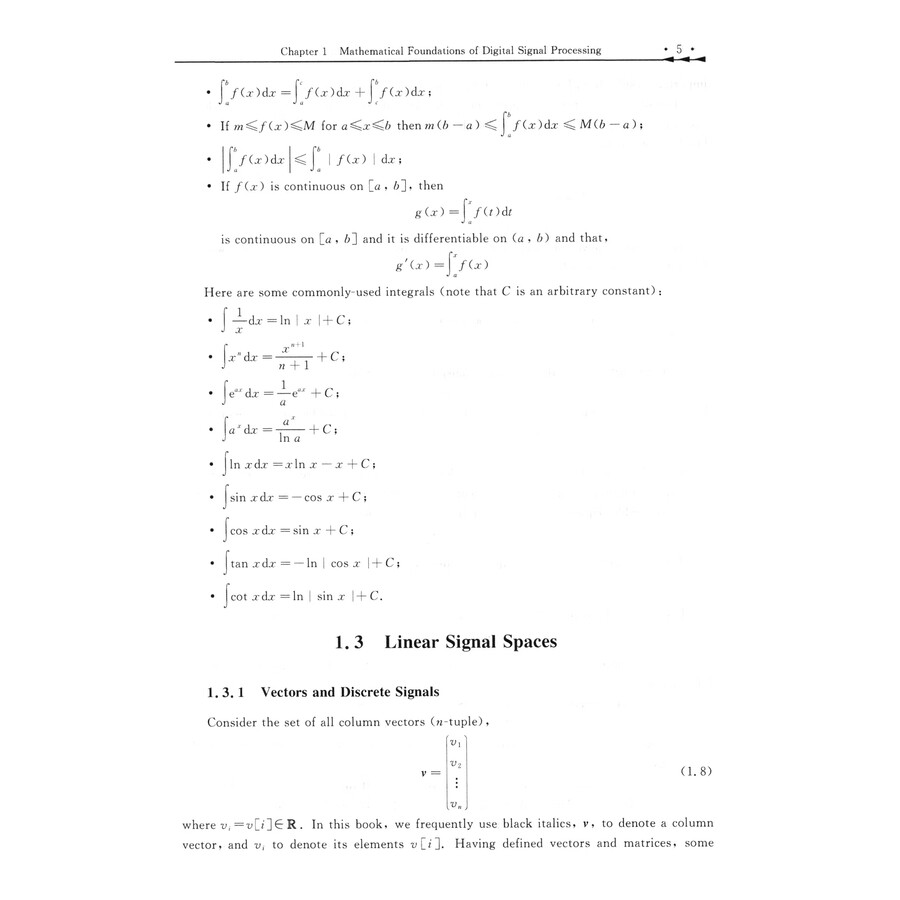FEEDBACK

#### Advanced Digital Signal Processing: From Theory to Applications

Price: \$16.92 \$11.89 (Save \$5.03)
Only 4 left in stock, order soon.

##### Details
This book is intended as an introduction to digital signal processing, ranging from basic theory to state-of-the-art processing algorithms.The first two chapters give a comprehensive review of foundations of digital signal processing.Chapters 3-6 are devoted to a discussion of basic signal processing theory, such as introduction in Chapter 3, transform in Chapter 4, discrete Fourier transform in Chapter 5 and Digital Filter Design in Chapter 6. Chapters 7-10 introduce advanced digital signal processing techniques, such as linear prediction in Chapter 7, Wiener filter in Chapter 8, estimator in Chapter 9 and detector in Chapter 10.By reading this book, you will find out that all these advanced signal processing algorithms are developed based on the basic signal processing theory and there is nothing mysterious and difficult.This book can be used both as a graduate textbook and as a reference book for self-study by practicing engineers and researchers.
##### Sample Pages Preview\$11.89